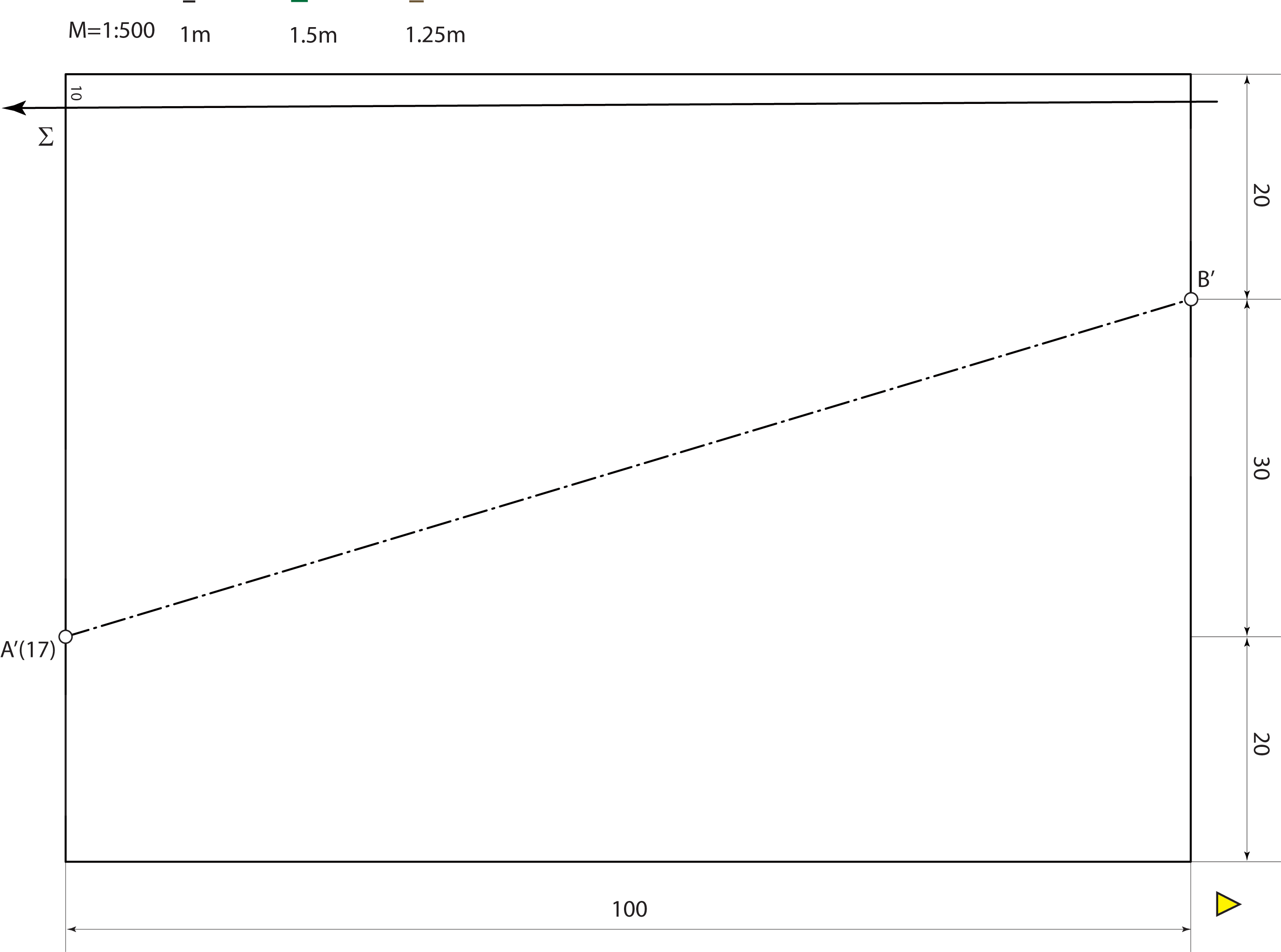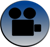### Straight inclined road on a ideal terrain

In cases where the subgrade is not horizontal, i.e. the road is inclined, the horizontals of the fills and cuts are not parallel with the edge of the subgrade (points on the boundary have different elevations). Therefore, the steps of solving the earthwork for building an inclined road are the same, but the planes of cuts and fills are different than the ones for a horizontal road.

In these cases, it will be used the earlier explained construction for the construction of the fills and cuts. (REMAINDER)

EXERCISE 5: Let the plain $$\Sigma$$ be the ideal terrain with the slope 20%. On the template this plane is given with the scale of slope and the horizontal with the elevation 10.
The road is given with its axis AB, has the width 10m and has the slope of 5% from point A (with the elevation 17) to the point B.
Solve the earthwork for building this road.

1st solution - elevated projectionSteps of the construction:
• Construct the horizontals of the plane $$\Sigma$$, $$i_\Sigma=5m$$.
• Construct the boundary line of the subgrade, grade the axis AB, and construct the horizontals of the subgrade (interval of the subgrade plane is 20m)
• Determine the neutral line which is the intersection of the subgrade and the ideal terrain.
• Construct one horizontal of the fills. This is the plane of the slope $$n_N=\frac{2}{3}$$, or interval $$i_N=\frac{3}{2}$$ which contains the edge of the subgrade.
Find the scales of slope for both fills, construct the horizontals of those planes and construct the intersection those planes with the terrain.
• Construct one horizontals of the cuts. This is the plane of the slope $$n_N=\frac{4}{5}$$, or interval $$i_N=\frac{5}{4}$$ which contains the edge of the subgrade.
Find the scales of slope for both cuts, construct the horizontals of those planes and construct the intersection those planes with the terrain.

2nd solution - program Rhinosurface of the terrain, the graded axis, subgrade and the neutral linefills and cutsfinal image 1final image 2

Created by Sonja Gorjanc, translated by Helena Halas and Iva Kodrnja - 3DGeomTeh - Developing project of the University of Zagreb